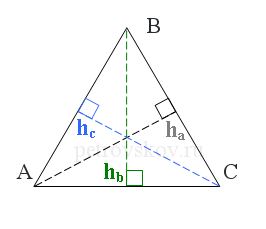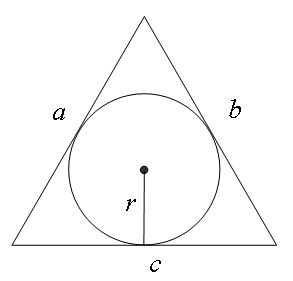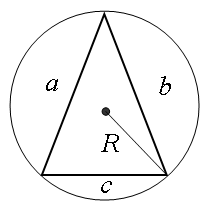# Triangle problem solving calculator

• ### Корзина

Пусто

Given a triangle ΔABC,
a = BC, b = AC, c = AB are sides of the triangle,A =CAB,B =ABC,C =BCA are angles of the triangle.How to use the triangle calculator. Please provide 3 values: a side and 2 other values (for example, an angle and a side, 2 angles or 2 sides). Then please provide "text from the image". Click "Calculate".

## Triangle calculator online

Sides

The triangle calculator finds solutions for the math problems – angles, and lengths of sides.

Solving a triangle means to find all its angles and sides. This calculator finds all elements of a triangle with angles 45 45 90, 30 60 90.

## How to solve a triangle

Using this online calculator, a triangle can be solved if given three, two sides and angles, by the law of sines and cosines, that is, it shows how to find the angles of a triangle for units as follows: degrees, radians, pi values.

The problem answer is calculated automatically by a computer program online, quickly, and conveniently.

This free triangle calculator provides formulas, detailed and accurate solutions, and answers to problems using the laws of sines and cosines.

The solution shows in detail how to find a third side if given two sides and the angle between them, or how to calculate two sides of a triangle if one side is given.

## Examples of problem solutions

1) Solve the triangle if given two sides and the opposite angle, i.e. the angle between them. The sides are given a = 12 cm, b = 8 cm, angle C = 60°. In order to solve a problem, it is required to provide the given values of the problem in an online form on this page. In the “a” field is to provide 12, in the “b” is to provide 8, in the “C” angle field is to provide 60. Click the “Calculate” button.

Result:
Side c = 10.58 cm,
Angle A = 79.1066° = 79°6'24'' = 79°6' = 0.4395π = 1.3807 rad
Exterior angle CAE of the angle A = 180° - 79.1066° = 100.8934° = 100°53'36'' = 100°54'
Angle B = 40.8934° = 40°53'36'' = 40°54' = 0.2272π = 0.7137 rad
Exterior angle ABF of the angle B = 180° - 40.8934° = 139.1066° = 139°6'24'' = 139°6'
Angle C = 60° = π/3 = 1.0472 rad
Exterior angle BCD of the angle C = 180° - 60° = 120°
Perimeter = 30.58 cm,
Semiperimeter = 15.29 cm,
Area = 41.57 cm2,
Height ha = 6.93 cm,
Height hb = 10.39 cm,
Height hc = 7.86 cm,
Median ma = 7.21 cm,
Median mb = 10.58 cm,
Median mc = 8.72 cm,
So the angle of the triangle was calculated by two sides and the angle.

2) how to find the angle of a triangle, knowing its sides or solve a triangle on three sides. Three sides are given a = 2 cm, b = 3 cm, c = 4 cm. In the “a” field of the online form is to provide 2, in the “b” field is to provide 3, in the “c” field is to provide 4. Click the “Calculate" button.

Using the law of cosines, we get
Angle A = 28.955° = 28°57'18'' = 28°57' = 0.1609π = 0.5054 rad
Exterior angle CAE of the angle A = 180° - 28.955° = 151.045° = 151°2'42'' = 151°3'
Angle B = 46.5675° = 46°34'3'' = 46°34' = 0.2587π = 0.8128 rad
Exterior angle ABF of the angle B = 180° - 46.5675° = 133.4325° = 133°25'57'' = 133°26'
Angle C = 104.4775° = 104°28'39'' = 104°29' = 0.5804π = 1.8235 rad
Exterior angle BCD of the angle C = 180° - 104.4775° = 75.5225° = 75°31'21'' = 75°31'
Perimeter = 9 cm,
Semiperimeter = 4.5 cm,
Area = 2.9 cm2,
Height ha = 2.9 cm,
Height hb = 1.94 cm,
Height hc = 1.45 cm,
Median ma = 3.39 cm,
Median mb = 2.78 cm,
Median mc = 1.58 cm,
So all the angles of the triangle were calculated.

3) solve a triangle by two angles and a side. In triangle ABC, side a = 5 cm, two angles B = 30°, C = 45°.
Side b = 2.59 cm,
Side c = 3.66 cm,
Angle A = 105° = 0.5833π = 1.8326 rad
Exterior angle CAE of the angle A = 180° - 105° = 75°
Angle B = 30° = π/6 = 0.5236 rad
Exterior angle ABF of the angle B = 180° - 30° = 150°
Angle C = 45° = π/4 = 0.7854 rad
Exterior angle BCD of the angle C = 180° - 45° = 135°
Perimeter = 11.25 cm,
Semiperimeter = 5.63 cm,
Area = 4.58 cm2,
Height ha = 1.83 cm,
Height hb = 3.54 cm,
Height hc = 2.5 cm,
Median ma = 1.95 cm,
Median mb = 4.19 cm,
Median mc = 3.54 cm,

## TrianglesA triangle is a polygon that consists of three points connected by three line segments. The three points of this polygon are vertices of the triangle, and the segments are sides or edges of the triangle. The drawing shows the triangle ΔABC, where A, B, C are its vertices, and AB, BC, AC are its sides. The angle at the vertex A is formed by the sides AB and AC. This angle denoted as the angleCAB.

There are different types of triangles. The type of the triangle depends on its angles and sides.

An equilateral or regular triangle consists of three equal sides and three equal angles. All three angles in the equilateral triangle equal to 60 degrees.If two sides have the equal length in a triangle, then this is an isosceles triangle.
In the isosceles triangle, two equal sides are called the edges, and the third side is called the base of the triangle. The scalene triangle is a triangle with sides of different lengths.

Properties of isosceles triangles:
1) the angles at the base are equal,
2) if two angles are equal in a triangle, then this is an isosceles triangle,
3) a median, that connects the vertex with the base, is a bisector and height.

Equal sides in triangles are denoted by one, two or three tick marks. Equal angles are denoted by one, two or three arc lines.

There are acute, obtuse, and right triangles.A triangle is right if one of the three angles of this triangle is equal to 90 degrees. The side opposite the angle of 90 degrees is called the hypotenuse. The hypotenuse is the longest edge in a right-angled triangle.
If a triangle is not right-angled, so this triangle is oblique and can be either obtuse or acute.

An obtuse triangle is a triangle in which one of the angles is greater than 90 degrees.

An acute triangle is a triangle in which all three angles are less than 90 degrees.

## Triangle Properties

In a triangle, only one angle can be greater than 90 degrees.

In a triangle, the sum of the angles is 180 degrees.

The exterior angle of the triangle is the adjacent angle at this vertex.
The calculation methods to find the exterior angle at the vertex:
a) sum two internal angles not adjacent to it,
b) calculate the difference between 180 degrees and the internal angle of this vertex.

The sum of any two triangle’s sides is always greater than the length of the third side.## Median and height

The median of a triangle is a line segment drawn from a vertex of the triangle to the midpoint of the opposing side.The height of a triangle is a perpendicular line segment drawn from a vertex of the triangle to the opposing side.

A triangle has three medians.

## Radius of the inscribed circle

A circle inscribed in a triangle is a circle drawn inside the triangle.The radius of this circle (r) is a perpendicular drawn from the center of the inscribed circle to one side of the triangle.

The center of the inscribed circle is the intersection point of two bisectors and equidistant from each side of the triangle.

The area and perimeter of the triangle are used to calculate the radius of the inscribed circle.

The circumradius (R) is the radius of the circumscribed circle passes through the three vertices of the triangle.Exterior angleCAE of the angleCAB = angle A;
Exterior angleABF of the angleABC = angle B;
Exterior angleBCD of the angleBCA = angle C.